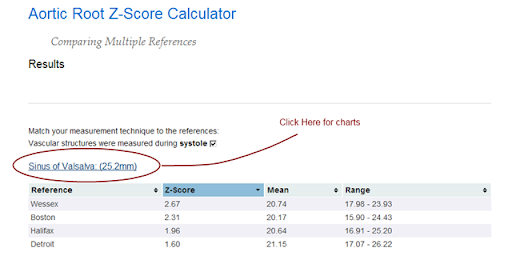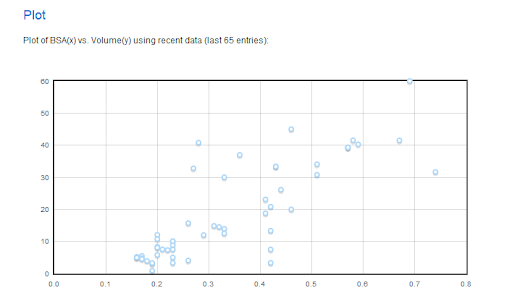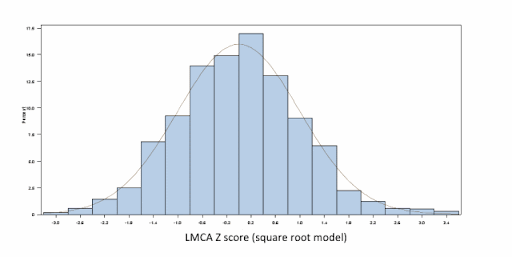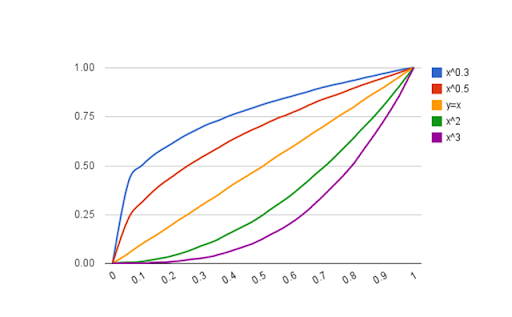CMR LVEDV Z-Score Mini-Smackdown

examining CMR references for LVEDV reveals interesting differences; doubt is cast upon the practice of generating z-scores for indexed values

I have been tinkering with z-scores for cardiac MRI and I thought it might be interesting to compare a couple of references for LV end-diastolic volume (I always think this stuff is interesting):

So, what I did was create some tables (using the mean and ± 2SD limits), generated some charts, and then made a series of z-score calculations over a range of LVEDV values for two hypothetical patients (view the spreadsheet and calculations for this data HERE).

Data:

First, the Alfakih data: based on their published values for “younger men” using SSFP, the LVEDVi is 87.6 ± 15.6.

LVEDV Reference Values: Alfakih et al.
BSA (m2) ULN (ml) Mean (ml) LLN (ml)
0.5 59 44 29
0.6 71 53 34
0.7 83 61 40
0.8 95 70 46
0.9 106 79 51
1.0 118 88 57
1.1 130 96 63
1.2 142 105 68
1.3 154 114 74
1.4 166 123 80
1.5 177 131 86
1.6 189 140 91
1.7 201 149 97
1.8 213 158 103
1.9 225 166 108
2.0 236 175 114

And then the Buechel data: based on their allometric equation, a * BSAb, and their published values for boys: a = 77.5, b = 1.38, and using the z-score form of... and their published value for the “SD” = 0.0426

LVEDV Reference Values: Buechel et al.
BSA (m2) ULN (ml) Mean (ml) LLN (ml)
0.5 36 30 25
0.6 47 38 32
0.7 58 47 39
0.8 69 57 47
0.9 82 67 55
1.0 94 78 64
1.1 108 88 73
1.2 121 100 82
1.3 135 111 92
1.4 150 123 101
1.5 165 136 111
1.6 180 148 121
1.7 196 161 132
1.8 212 174 143
1.9 229 188 155
2.0 245 201 166

Charts:Z-Scores:

Generated Z-Scores for Patient BSA = 0.7
LVEDV Z: Alfakih Z: Buechel
15 -4.3 -11.7
20 -3.9 -8.8
25 -3.4 -6.5
30 -2.9 -4.7
35 -2.5 -3.1
40 -2 -1.7
45 -1.5 -0.5
50 -1.1 0.6
55 -0.6 1.5
60 -0.1 2.4
65 0.3 3.2
70 0.8 4
75 1.3 4.7
80 1.7 5.3
85 2.2 6
90 2.7 6.5

Generated Z-Scores for Patient BSA = 1.4
LVEDV Z: Alfakih Z: Buechel
50 -3.4 -9.2
60 -2.9 -7.3
70 -2.5 -5.8
80 -2.0 -4.4
90 -1.5 -3.2
100 -1.1 -2.1
110 -0.6 -1.2
120 -0.1 -0.3
130 0.3 0.5
140 0.8 1.3
150 1.3 2.0
160 1.7 2.7
170 2.2 3.3
180 2.7 3.9
190 3.1 4.4
200 3.6 4.9

Summary

Buechel et al. sum it up nicely in their discussion:

cardiac volumes have a non-linear relation to body surface area, and since the exponential values are different for different cardiac parameters, it would not be appropriate to provide normal values simply indexed to BSA

The textbook Echocardiography in Pediatric and Congenital Heart Disease has an excellent and thorough description of the practice of “indexing”. Essentially, the problem boils down to this: for LVEDV, none of the assumptions for the relationship are met:

In order for the per-BSA method of indexing to work, three assumptions must be met. The relationship to BSA must be linear, the intercept of the regression must be zero, and the variance must be constant over the range of BSA.

If you had to choose a reference for LVEDV in children measured with cardiac MRI, I would have to wonder why anyone would not use the data from Buechel et al.— unless they just did not have those calculations handy.

Well, now they do:

BSA Methods and Cardiac Z-Scores

A spreadsheet comparing different BSA calculations on various patients-- and the resulting BSA-adjusted z-scores-- reveals negligible differences.

Can we compare z-scores from various references when the methods for calculating BSA are different? How?

If a given group of z-score equations are BSA adjusted, and a given patient has a different BSA depending on the BSA formula, how do you perform a comparison? Meaning, if equation A uses BSA formula x, and equation B uses BSA formula y, to what extent are differences in the z-scores due to differences in BSA?

Which then spawns these questions:

• What is a clinically important difference in BSA?
• a tenth of a meter2?
• a hundredth?
• a thousandth?!?
• How many significant digits are important when comparing z-scores?

I made this spreadsheet in an effort to examine some of these questions.

(the example z-score equation is from Kaiser et al., JCMR 2008.)

Looking over this data, I totally agree with Dallaire and Dahdah, JASE 2011, who noted:

There was virtually no difference when Z-score equations were derived from BSA estimated with different equations, and misclassification was rare.

Estimating Pulmonary Artery Pressure from Acceleration Time

Calculate PA pressure using pulmonary artery Doppler acceleration time.

Methods

Doppler interrogation of the pulmonary artery was performed in the parasternal short axis view, with the pulsed-wave sample volume placed at the annulus of the pulmonary valve. The acceleration time was defined as the interval between the onset of systolic pulmonary arterial flow and peak flow velocity.

Aortic Root Reference Values Charts

A little decoration for the aortic root z-score calculator: charts.

Charts add a little ‘sugar’ to the reporting of the aortic root z-scores, by adding an important visual element to the situation.

I have tinkered with charts for the aortic root before, with a few ‘smack-down’ pages, but this is the first large-scale incorporation of the charts with the z-score calculator (well, that’s not exactly true, I do have a cute little chart for the TAPSE z-score calculator. But as far a scale , goes-- this is bigger and better.)

Now, when you see the results page from a z-score calculation, you can click on the sub-heading to link to a page with the appropriate charts for that patient:Clicking on the link illustrated in the above image takes you to these charts:

http://aoroot.parameterz.com/chart?site=sov&bsa=0.99&score=25.2

I took a small bit of design license in building these chart pages: I use the Haycock BSA formula for the patient’s BSA, even though it is not always (rarely?) the default equation used for the individual references. It made the difference between doing it in one sitting (OK, two), and probably not getting it done at all. It was just too complicated the way I had designed the base classes.

Aortic Root and LV Volume Z-Score Calculators

Two new z-score calculators on Parameterz.com plus a paradigm shift or two

First, the z-score calculators:

1. LV End-Diastolic Volume z-scores, using the 5/6 A-L method
2. A consolidated Aortic Root z-score calculator

The paradigm shifts are these:

1. All calculations are now server-side, using Python
2. The Aortic Root calculator is a prototype for combining all available references into one calculator/results page

LV EDV Z-Score Calculator

These z-scores are based on the recent work Normal values for left ventricular volume in infants and young children by the echocardiographic subxiphoid five-sixth area by length (bullet) method, from the Feb 2011 JASE. This is a terrific approach to what is traditionally considered a difficult measurement to calculate and normalize. For one, ventricular volumes calculated by the traditional Simpson’s bi-plane technique are notoriously unreliable. To wit, the recent abstract from the PHN citing the 5/6 A-L technique as superioir:

Variability of LV size and function measurements in children with DCM is reduced when utilizing the 5/6 AL algorithm.

(Margossian et al., JASE; May 2010)

The authors cite their own previous work as demonstrating the 5/6 A-L technique to be valid with regards to correlating well with MRI volumes.

On another front, the equations presented in this article also dramatically simplify the very complex relationship of ventricular volume and size, thought to be multi-factorial based on ejection fraction, heart rate, cardiac output, and BSA. A theoretical allometric relationship between EDV and BSA was recently described to be approximately

...the predicted relationship is EDV ≈ BSA1.4

Theoretical and empirical derivation of cardiovascular allometric relationships in children.
Sluysmans T, Colan SD.
J Appl Physiol. 2005 Aug;99(2):445-57

The calculator itself is sort-of a hybrid calculator, in that the BSA and volumes are calculated with JS on the client, then the page is posted to the server and the z-scores are calculated using Python. Another novel aspect to this calculator is that, by virtue of the calculations being performed on the server, I can provide a running tab of recent calculations, resulting in a real-time plot of actual EDV vs. BSA data:This is not the right calculator to be used for collecting normative data, but what I am learning about server-side programming-- like with this calculator-- is getting me much closer to realizing such a design.

Aortic Root Z-Score Calculator

This calculator is a combination of what I had learned from building the LVEDV calculator and from the prototype design of the multi-referenced coronary artery z-score calculator. The essence of the design is that the patient demographics and measurement data are entered once and the z-scores and reference ranges from each of several references are returned to you in what I hope is an easy  to use, sortable, results table:

http://aoroot.parameterz.com/calc?ht=128&wt=28&aov=15.5&sov=25&stj=18&aao=21

The data required to make the calculations are part of the url (i.e., the “query string”) so the results are essentially permanent and “linkable” should anyone find any need for such a feature.

The references for calculating aortic root z-scores use various BSA equations. This calculator applies the correct BSA formula to each calculation, and thus the z-scores between each reference should be comparable, even though the calculated BSA’s are slightly different. For posterity’s sake, the different BSA calculations are also provided, which therefore makes this a very elaborate BSA calculator:

http://aoroot.parameterz.com/calc?ht=128&wt=28

Also, since not all the references use the same measurement methodology, an allowance is made to show only the appropriate calculations based on your local methodology, i.e., I filter the results based on whether the user indicates the measurement of the vascular aspects of the aortic root were measured in systole or diastole.

To be sure, all of this could have been done on the client with JavaScript, but I thought it was time to grow up.Reference Benchmarks

A look at evolving benchmarks for pediatric cardiac reference data

I read with some interest the article Optimal Normative Pediatric Cardiac Structure Dimensions for Clinical Use and was amused to see an off-hand reference to Parameter(z):

Pettersen et al’s paper does not specify which BSA formula was utilized; however, the cardiac dimensions they measured have been normalized using both the DuBois and the Haycock et al formula and are available elsewhere.

I am always curious about the context in which Parameter(z) might appear, and in this case I’d like to add my own two cents.

The authors of the paper set out to review the literature and recommend the “optimal normative data set” for cardiac structures/dimensions-- a familiar, if not worthy, cause. Along the way the authors note several criteria (I am going to call them benchmarks)  for consideration:

• sample size—larger studies are better
• normalization factor
• allometric equation is best
• Haycock BSA equation is best
• measurement technique/protocol—those according to current guidelines are best
• consideration for race and gender
• “sophisticated” analysis (like the LMS method) are mentioned (preferred?)

Race and gender considerations are mentioned, but it is not clear if these were separate criteria, or if they only had a bearing on which BSA formula to use. To give them the benefit of the doubt, I will leave them in as bonus benchmarks. The authors then go on to recommend the data from Detroit as the “optimal” data set.

While I don’t disagree with any of these benchmarks, I do think some clarification might be useful. An allometric equation is certainly a useful approach for describing the growth of structures-- the biologic relationship between structure and body size-- and I am a big fan of this approach. A “sophisticated” analysis like the LMS method is a completely different approach, independent and ignorant of any underlying biologic process. I am also a big fan of this type of analysis. The message here is that you either do a predictive analysis, preferably using an allometric equation OR you do a descriptive analysis, preferably using the LMS method.

The principle feature of the LMS method is that it accounts for things like skew and heteroscedasticity and results in valid, normally distributed z-scores. There are other ways to achieve this though, as was recently present in the manuscript New equations and a critical appraisal of coronary artery Z scores in healthy children. Here, the authors apply the Anderson-Darling goodness-of-fit test to determine if their data (derived from an allometric equation) depart from a normal distribution.

So, the first point is this—one of the benchmarks should read: equations result in valid, normally distributed data, either by use of the LMS (or similar) method, or by performing some type of analysis confirming a normal distribution.

The second point is this: I don’t think the Detroit data holds up well to these benchmarks and should not be described as “optimal”. Certainly, theirs is a large study (>700 patients), and they indeed followed current guidelines for the measurements. However, on every other point I believe they fail:

• they do not use an allometric equation (theirs is a polynomial equation)
• they do not use the preferred BSA equation (via a personal communication, I learned they used DuBois & DuBois)
• they do not include race or gender in their analysis (in fact, no demographic data is presented—at all)
• they did not use the LMS method, or perform any distribution analysis

Are they better than nothing? Absolutely
A step in the right direction? Agreed
But optimal?

I will say this about the Detroit z-score calculator though: it is the most popular of all the calculators at Parameter(z). In the past 6 months:

• 19,165 pageviews; 15.26% of all site traffic
• average 160 visits per day
• average 3:56 time on page
• visited most by users in California, Virginia, Georgia, Chile, and North Carolina

The equations may not be optimal, but—for better or worse—they are getting a lot of use.

Q and A with Frédéric and Nagib

The January issue of JASE holds this gem of a manuscript:

New equations and a critical appraisal of coronary artery Z scores in healthy children.
Dallaire F, Dahdah N.
J Am Soc Echocardiogr. 2011 Jan;24(1):60-74. Epub 2010 Nov 13.

The title couldn’t be any more fitting-- it is a must-read for anyone interested in the matter of z-scores for pediatric cardiology.

The authors have graciously agreed to allow me to post their answers to my “follow up  questions”:

Q: Thanks for introducing us to the Anderson-Darling normal distribution test. However, why not include a few frequency vs. residuals histograms for the cavemen in the audience like me? We like pictures...
A: We used such histograms in our analysis to “visually” assess normality. Our article was however long and we had to cut down some of the text and figures. Here’s the frequency distribution for left main coronary artery Z scores (final model with square root of body surface area)Q: Are there other normality tests? Why the Anderson-Darling test?
A: The Anderson-Darling test tests whether a sample fit to a given distribution. When used to test for departure from normality, it is one of the most powerful. SAS also gives the results for the Kolmogorov-Smirnov and Cramer-von Mises tests, which are less sensitive.
Q: The power model described in the article has the form: y = a +b1x2+b2x Why isn't that a polynomial model (quadratic)? I was expecting a model of the form: y : x(see chart).Is this just a matter of semantics, or is the power model misnamed?
A: Yes, we should have named it a polynomial model.
Q: Judging by the spread/skew of the +/- 2SD curves on the “exponential model”, it looks like a log-normal curve... how did you treat the SD with this model?
A: In the exponential model, body surface area and coronary diameter were both log-transformed and then the model was fitted. The SD used was thus the one of a linear model on log-transformed values. Even with the logarithmic transformation, there was residual heteroscedasticity and a weighted least-square model was used. The weight in the model was the inverse of the linear regression of the residuals (on log-transformed values).
Q: Your “exponential model” empirically arrived at an exponent of 0.544-- similar to your theoretical “square root model”: y = a + b1x0.5. However, the Boston and Washington, D.C. models are similar and have exponents of something like 0.3xx. Is the difference between the exponents attributed to your larger sample size, or could there be something else going on here?
A: Hard to say. I would guess that aside from the greater sample size, it is likely the better representation of small children and infants that made the difference. If the theoretical model of optimal cardiovascular allometry proposed by Sluysmans and Colan in 2004 is true, it seems logical that a good representation of children from all ages helped to produce a “real-life” model close to the theoretical model proposed by Sluysmans and Colan.
Q: The final models described in the article are very similar in form to many of the z-score equations from Boston: 2 regression equations; one for the mean, one for the SD. However, the Boston equations predict the SD by a regression against BSA. Your SD equations are run against the square root of the BSA. How does one determine the best model for the variance?
A: The best model for variance should be determined in the same way the model for the mean is. That is, one should ensure that the residuals are free of a trend (no association should exist between the residual and the independent variable). In other words, there should not be an association between the residuals of the residuals and the dependent variable. This was verified for our data but was apparently not done in previous series, including Boston’s.
Q: I keep wondering: if we took a hundred patients with the same BSA, what is the mean/median/mode/min/max/distribution of those measurements? What does that curve look like? Had you considered using something like the “LMS” method to do a group-wise evaluation?
A: The use of a Z score assumes that the coronary diameters of x patients with a given BSA are normally distributed. In fact, our modelisation supposes that for any given value of BSA, there exists a number of subjects that are normally distributed around the mean (see our figure). If so, the median, mode and mean should be the same. The important thing is that in the absence of such a distribution, the Z score cannot be used to estimated percentiles, which is its principal (only?) value. In the lab, one wants to know if a coronary diameters exceeds the 95th or 98th percentile for a given BSA to be able to answer the question “is that coronary abnormal?”. Such percentiles can only be estimated if the data is normally distributed.Q: What do you think explains how the proximal RCA has a normal distribution in your analysis but the distal RCA does not?
A: The distal RCA has two particularities. 1) distal RCA is the most difficult to view and, therefore, to measure. We are confident all distal RCA measurements we use to compute our equations were properly imaged and measured (number of distal RCA samples are the lowest compared to the other segments in our report). The difficulty of obtaining good image might have played a part, but we do not think this is the main reason for the non normal distribution... 2) Essentially, the size of the distal RCA depends on whether or not the RCA is dominant or not (typically 2/3 vs 1/3 of normal humans). We think this is the most probable explanation for not perfectly symmetric variability among subjects. In brief, we believe that the dominance factor is the key answer. One way to verify this hypothesis is to take the adventure of measuring the distal circumflex (posterior rim) and compare with the distal RCA in a series of subjects...
Q: If you consider coronary arteries as a microcosm of the larger reference values issue in pediatric cardiology, what implications does your work have for the existing body of z-score equations?
A: Surprisingly, very little proper validation of reference values and normalisation has been done in paediatric echocardiography. We advocate for a close examination (and potentially a redo when appropriate) of nearly all equations so far available in the literature. Some of them are probably adequate, but in the absence of a good description of the final distribution, it is difficult to affirm with confidence.
Q: Do you have any advice for others, like the ASE, that are going forward with developing new models and z-score equations?
A: Simply fitting a modelled curve in the data is not enough. Since Z scores are dependent on the distribution of the data, one should absolutely test the Z score distribution obtained. One should also ensure that there is no residual trend and no residual heteroscedasticity. We believe that Z scores are a very useful tool for interpreting cardiac structure dimensions in paediatric settings. They must however be based on sound unbiased mathematical grounds.

Naturally, a z-score calculator has been posted up at ParameterZ.com:

http://parameterz.blogspot.com/2010/11/montreal-coronary-artery-z-scores.html

I also made this into it’s own project, making comparisons between the new data and previous coronary artery z-score equations: